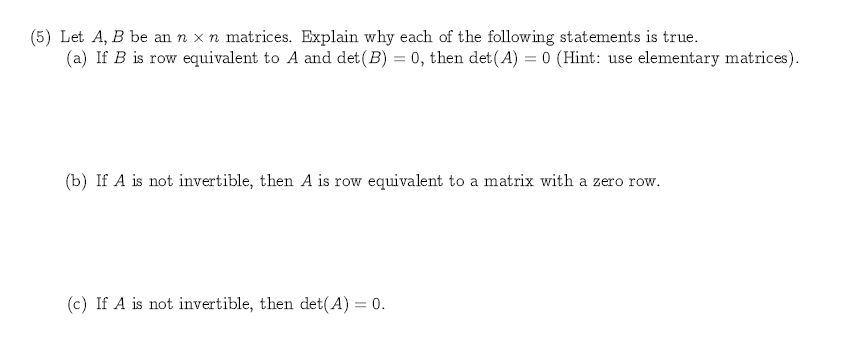# (5) Let A, B be an n x n matrices. Explain why each of the following statements is true.(a) If B is row equivalent to A and det (B) 0, then det(A) 0 (Hint: use elementary matrices)(b) If A is not invertible, then A is row equivalent to a matrix with a zero row(c) If A is not invertible, then det(A) 0

Question
1 viewshelp_outlineImage Transcriptionclose(5) Let A, B be an n x n matrices. Explain why each of the following statements is true. (a) If B is row equivalent to A and det (B) 0, then det(A) 0 (Hint: use elementary matrices) (b) If A is not invertible, then A is row equivalent to a matrix with a zero row (c) If A is not invertible, then det(A) 0 fullscreen
check_circle

Step 1

5.

(c).

Note that, a matrix is singular if and o...

### Want to see the full answer?

See Solution

#### Want to see this answer and more?

Solutions are written by subject experts who are available 24/7. Questions are typically answered within 1 hour.*

See Solution
*Response times may vary by subject and question.
Tagged in

### Math